# 2. A dragon is flying around in a pattern given by the parametric curve r(t) (cos(t) cos((sin(t) sin(t) cos(t)j. cos(t) - cos sin(t)-sin(t) cos(t))j (a) Find a formula for the velocity of the dragon...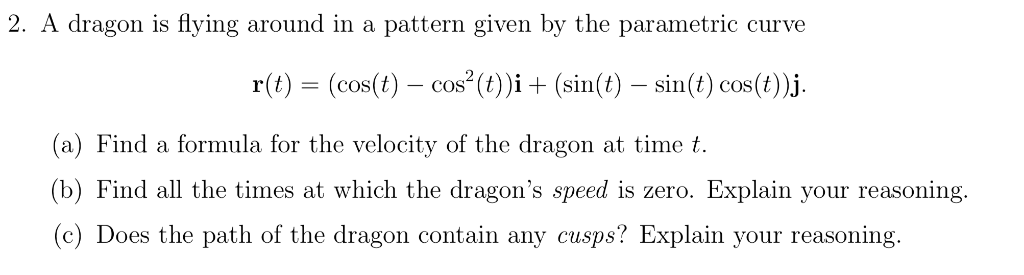2. A dragon is flying around in a pattern given by the parametric curve r(t) (cos(t) cos((sin(t) sin(t) cos(t)j. cos(t) - cos sin(t)-sin(t) cos(t))j (a) Find a formula for the velocity of the dragon at time t (b) Find all the times at which the dragon's speed is zero. Explain your reasoning. c) Does the path of the dragon contain any cusps? Explain your reasoning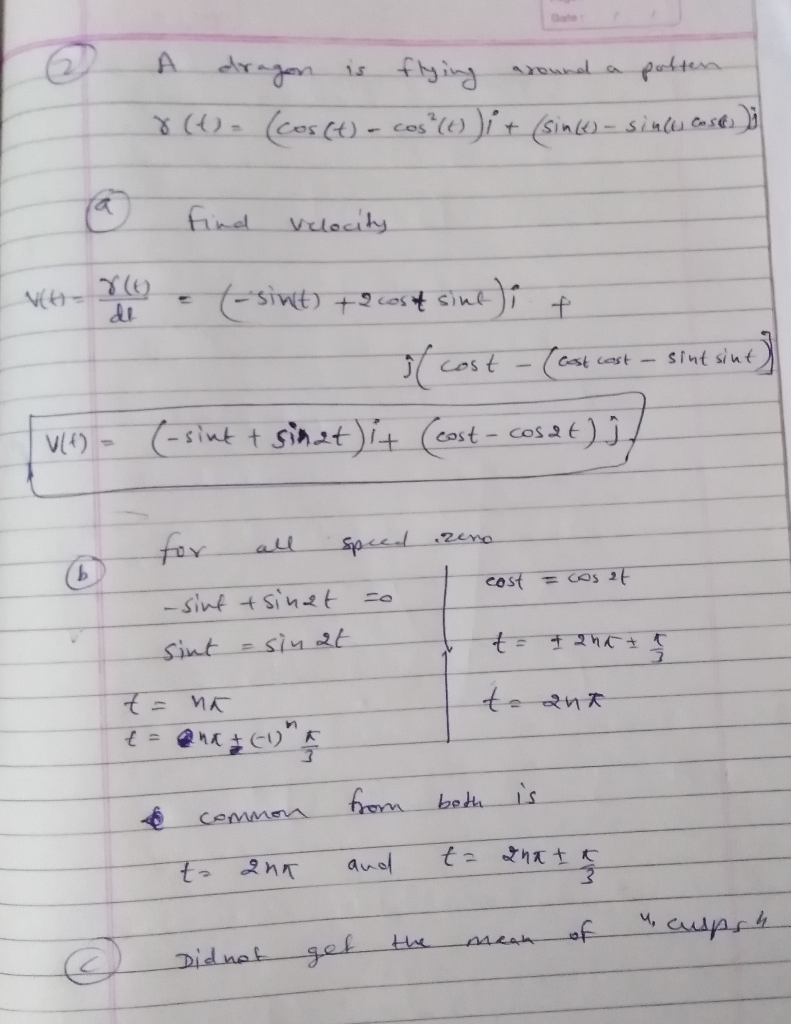##### Add Answer of: 2. A dragon is flying around in a pattern given by the parametric curve r(t) (cos(t) cos((sin(t) sin(t) cos(t)j. cos(t) - cos sin(t)-sin(t) cos(t))j (a) Find a formula for the velocity of the dragon...
Similar Homework Help Questions
• ### show work please 密38. Graph the curve with parametric equations r-sin y sin 2t, z cos 4. Explain its shape by graphing...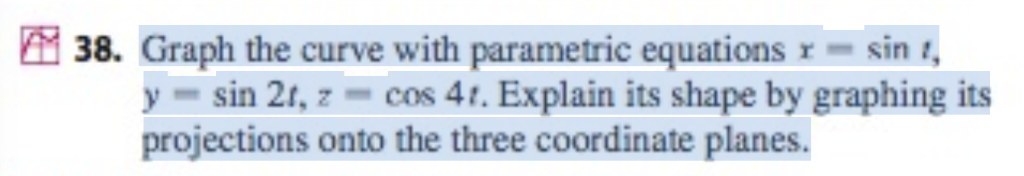show work please 密38. Graph the curve with parametric equations r-sin y sin 2t, z cos 4. Explain its shape by graphing its projections onto the three coordinate planes. 密38. Graph the curve with parametric equations r-sin y sin 2t, z cos 4. Explain its shape by graphing its projections onto the three coordinate planes.

• ### Consider the parametric curve given by x(t) = 16 sin3(t), y(t) = 13 cos(t) − 5 cos(2t) − 2 cos(3t...

Consider the parametric curve given by x(t) = 16 sin3(t), y(t) = 13 cos(t) − 5 cos(2t) − 2 cos(3t) − cos(4t), where t denotes an angle between 0 and 2π. (a) Sketch a graph of this parametric curve. (b) Write an integral representing the arc length of this curve. (c) Using Riemann sums and n = 8, estimate the arc length of this curve. (d) Write an expression for the exact area of the region enclosed by this curve.

• ### Find dy/dx for the curve given by x= -sin(t) and y= cos(t)

Find dy/dx for the curve given by x= -sin(t) and y= cos(t)

• ### Find the length of the parametric curve x=cos(cos(2t)),y=sin(cos(2t)) for 0

Find the length of the parametric curve x=cos(cos(2t)),y=sin(cos(2t)) for 0<

• ### Given the parametric equation x = t ¨C sin t and y = 1 ¨C cos t B

. Given the parametric equation x = t ¨C sin t and y = 1 ¨C cos t B. Find the points (x, y) on the curve where the tangent is horizontal or vertical. Label which.6. Find the area of the region bounded by x = sin t, y = e^(2t), 0 ¡Ü t ¡Ü (pi/2).8. Find the surface area generated by rotating the curve x = 4e^(t/2) and y = t - e^t for 0 ¡Ü t ¡Ü 1.9....

• ### (a) Let θ : R-+ R be a smooth function. Find the (signed) curvature of the curve a:R- R2 given by cos(θ(t)) dt,I α(s) sin(θ(t)) dt Use your result to give another geometric interpretation to the (...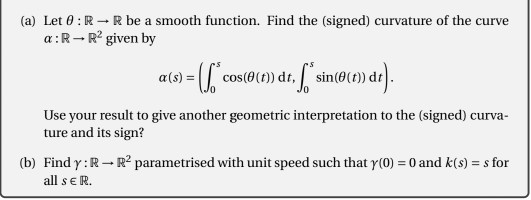(a) Let θ : R-+ R be a smooth function. Find the (signed) curvature of the curve a:R- R2 given by cos(θ(t)) dt,I α(s) sin(θ(t)) dt Use your result to give another geometric interpretation to the (signed) curva- ture and its sign? to) rindy,R-- parmetrised with unit speed suchhat y -0and kt) - s for all seR. (a) Let θ : R-+ R be a smooth function. Find the (signed) curvature of the curve a:R- R2 given by cos(θ(t)) dt,I...

• ### marks] The position of a particle is given as a function of time by r(t)=(1-cos(27t)i+ (1-t)sin(2nt)j+ 4tk with i (1,0,...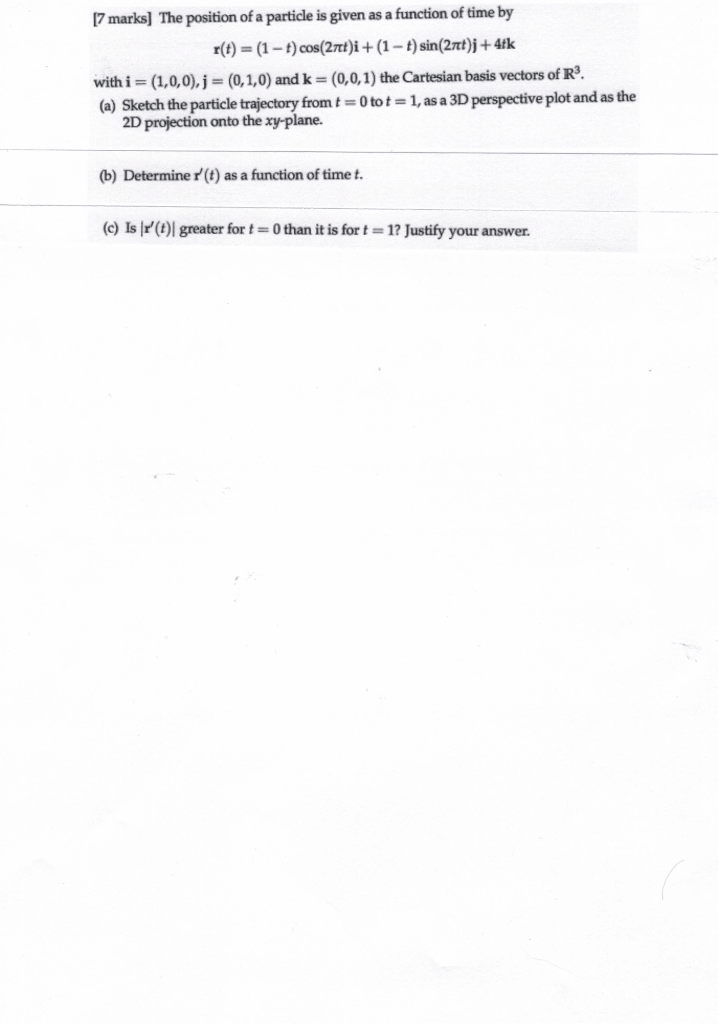marks] The position of a particle is given as a function of time by r(t)=(1-cos(27t)i+ (1-t)sin(2nt)j+ 4tk with i (1,0,0), j = (0,1,0) andk = (0,0,1) the Cartesian basis vectors of R3. (a) Sketch the particle trajectory from t 0 tot= 1, as a 3D perspective plot and as the 2D projection onto the xy-plane. (b) Determiner(t) as a function of time t. (c) Is r'(t) greater for t 0 than it is for t 1? Justify your answer. marks]...

• ### (a) Find the unit tangent vector, T(t) and the unit normal vector, N(t), for the space curve r(t) cos(4t), sin(4t), 3t...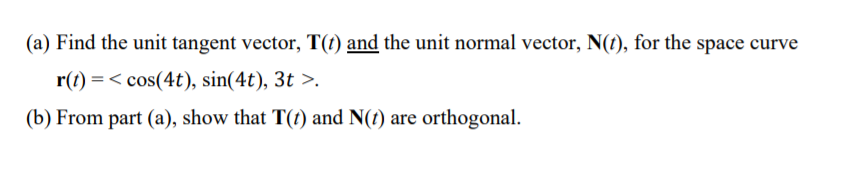(a) Find the unit tangent vector, T(t) and the unit normal vector, N(t), for the space curve r(t) cos(4t), sin(4t), 3t >. (b) From part (a), show that T(t) and N(t) are orthogonal (a) Find the unit tangent vector, T(t) and the unit normal vector, N(t), for the space curve r(t) cos(4t), sin(4t), 3t >. (b) From part (a), show that T(t) and N(t) are orthogonal

• ### a. Find the curvature of the curve r(t)- (9+3cos 4t)i-(6+sin 4t)j+10k. o. Find the unit tangent vector T and the principal normal vector N to the curve -π/2<t<π/2. r(t) = (4 + t)i-(8+In(sec...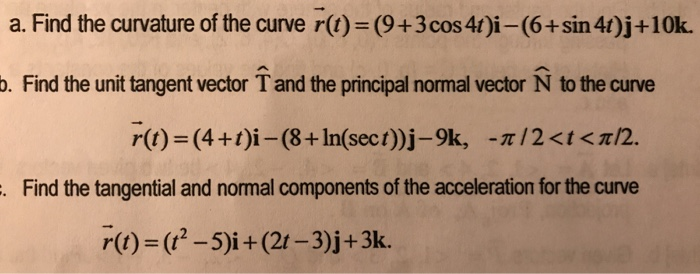a. Find the curvature of the curve r(t)- (9+3cos 4t)i-(6+sin 4t)j+10k. o. Find the unit tangent vector T and the principal normal vector N to the curve -π/2<t<π/2. r(t) = (4 + t)i-(8+In(sect))j-9k, Find the tangential and normal components of the acceleration for the curve r(t)-(t2-5)i + (21-3)j +3k. a. Find the curvature of the curve r(t)- (9+3cos 4t)i-(6+sin 4t)j+10k. o. Find the unit tangent vector T and the principal normal vector N to the curve -π/2

• ### Find the tangent line for the curve

Let r(t) =v2 cos t i+v2 sin t j+sin 5t k, 0= t = 2p.(a) Find scalar parametric equations for the tangent line tothe curve at the point (1, 1,-v2/2).

Need Online Homework Help?